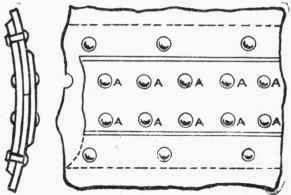The Boiler Inspection Department of Massachusetts recommends the following formula for determining the thickness of boiler plate:Fig. 161. - Butt Joint.Fig. 162. - Double-Riveted Butt Joint.

T = P X R X FS / TS X %

 Where T = thickness of the boiler plate in inches. P = boiler pressure in pounds per square inch. R = radius (½ diam. of boiler) in inches. FS = TS = tensile strength of the metal in pounds per square inch. % = strength of the joint.

The efficiency or strength of a joint is the percentage of the strength of the solid plate that is retained in the joint. It depends upon the kind of joint and method of construction. If the thickness of the plate is more than ½ inch, the joint should always be of the double-bolt type.

Example.-What thickness of plate should be used when making a 40-in. diameter boiler to carry 125 lbs. pressure, if the strength of the plate is 60,000 lbs. per square inch, using a factor of safety of 6, and 50% as the strength of joint?

T = 125 X 20 X 6 / 60,000 X .50 = ½ in. sheet

To find the safe working pressure use the following formula, where the letters have the same significance as in the previous formula:

P = TS X % X T / R X FS

Example. - Find the safe working pressure of the same boiler.

P = 60,000 X .50 X .5 / 20 X 6 = 125 lbs.